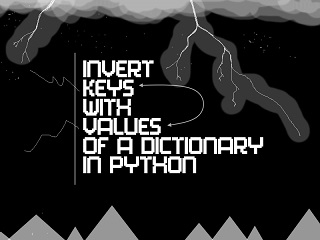# How to Reverse/Invert Dictionary Mapping in Python

Python dictionary is a powerful data structure used by developers to store data in key-value format. But sometimes you may need to reverse or invert the key-value mapping in python dictionary. In this article, we will learn how to reverse/invert dictionary mapping in python. This is often required when you are transferring data between two applications with different data requirements.

## How to Reverse/Invert Dictionary Mapping in Python

For our article, we have assumed that the values in original dictionary are unique. This is important since these values will be used as keys in inverted dictionary and dictionary keys need to be unique values.

Let us say you have the following dictionary.

`test = {'a': 1, 'b': 2}`

Let us say you want to obtain the following dictionary.

`inv_test = {1: 'a', 2: 'b'}`

You can easily invert the dictionary using dictionary comprehension, as shown below.

`inv_test = {v: k for k, v in test.items()}`

If you are using python 2+, then you will need to modify your command as shown below.

`inv_test = {v: k for k, v in test.iteritems()}`

You can also create a list of tuples where first element is the value and the second element is the key, and then call dict() function on the list to convert it into a dictionary.

`dict((v, k) for k, v in test.items())`

If you use Python 2+,

`dict((v, k) for k, v in test.iteritems())`

All the above examples have assumed that your dictionary values are unique. If values in test dictionary are not unique, then you will need to use the following approach. Let us say you have the following dictionary.

`test = {'a': 1, 'b': 2, 'c':2}`

As you can see, both keys b and c have same value 2.

```inv_test = {}
for k, v in test.items():
inv_test[v] = inv_test.get(v, []) + [k]```

If you are using Python 2+, you can use the following code.

```inv_test = {}
for k, v in test.iteritems():
inv_test[v] = inv_test.get(v, []) + [k]```

In the above code, items() and iteritems() return iterator to dictionary. We loop through the dictionary key-value pairs one by one, and in each iteration store value as the key and the keys as value. If there are more than one keys with same value, we store all those keys in a list, in the inverted dictionary. So here is the output we get when we run the above code. As you can see, [‘c’,’b’] is a list since both have the same value in original dictionary.

`{1: ['a'], 2: ['c', 'b']}`

In this article, we have learnt a couple of simple ways to invert a dictionary in python. You can use either of these methods as per your requirement. Here is the code to invert such as dictionary.